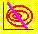5.8 Chapter 5: Exercises

1. Define an isomean curve.

2. Describe the shape of an isomean curve, and explain why it takes this shape.

3. Define an isovariance curve.

4. Describe the shape of an isovariance curve, and explain why it takes this shape.

5. In Chapter 5 of CAPM Tutor, start with the portfolio in which stocks 1 and 2 have zero weight, and stock 3 has weight equal to one. Now, change the weight of stock 1 continuously from zero to one, and observe the display change.

a) Explain what is being plotted in the return/standard deviation window.

b) Explain what is being plotted in the portfolio weights window in terms of the isomean and isovariance curves.

c) Provide a geometric description of the set of solutions that have been traced out in the portfolio weights window.

d) Describe the mathematical problem that allows you to solve for the portfolio weights in c).

e) Provide an interpretation of the point in the portfolio weights window that corresponds to the point at which the curve bends back in the return/standard deviation window.

previous topic

next topic